Search IntMath
Close

# Impossible integral question

By Murray Bourne, 10 Sep 2010

Can you help me with this question pls? Thx 🙂

Integration from 0 to -1
dx

this is what i manage to work out
let u = 2x^2+x
(du)^-2 = (4x+1)^-2 dx
1/2 int (u^2)/2 (du)^-2

then i'm stuck bcoz i dunno how to solve (du)^-2

Her question says: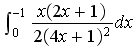Her last line actually means this: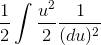Since Scarlett was willing to put in some effort to help herself, I replied:

Hi Scarlett

Good on you for showing your working! (Most people don't and I have no idea where they are having a problem).

Interesting try, but you need to make sure you end up with "du" (never (du)^2) and the "du" needs to be on top of the fraction (you should never have (du)^-2).

Now, before going into the solution, let's point out something important.

## Care with order of limits!

Scarlett wrote "Integration from 0 to -1".

This literally means the following (with the 0 as the lower limit of the integral and −1 as the upper limit).But if we reverse the order of the limits, our final answer will be the negative of the answer without reversed limits.

Since most school math text books have the smallest (left-most) number as the lower limit, I presumed her question actually meant the following (with −1 as the lower, left-most limit and 0 as the upper limit).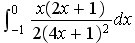This is the version we are going to solve.

This is a language issue, where we normally read top to bottom (Integration from 0 to -1) but we need to say integral limits from the bottom number to the top. It should have been Integration from -1 to 0.

Now, before I go on, have any of you spotted why I titled this article "Impossible integration question"?

## Possible approaches

When you come across a new integral, you often need to do a bit of trial and error until you get the right approach.

Like Scarlett, I initially thought it might be a substitution, but with the more likely choice for u being:

u = 4x + 1

This will give u2 on the bottom and du on top, but there is a function of x on top (not a "nice" number), so it looks like it might give trouble.

Perhaps there is an easier way.

## Partial Fraction approach

My next thought was to try partial fractions. This process allows us to split apart a fraction into its parts, thereby giving us a much simpler expression to integrate.

For simplicity, I first take the 1/2 out the front, remove it (and I need to remember to put it back at the end).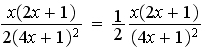Since we have a denominator that contains repeated linear factors, we are looking for an expression in the form: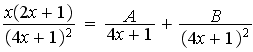However, you can try all day and never solve this for A and B, since we can only find partial fractions if the degree of the numerator (highest power of x on top) is less than the degree of the denominator (highest power of x on the bottom).

In this example, the degree of both top and bottom is 2, so we cannot use this approach to finding the partial fractions.

However, with a bit of magic, we can still find a partial fraction decomposition and solve our integral.

[Note: Math teachers often seem to perform "magic" by pulling things out of thin air in order to solve some problem. I'm able to perform the following trick because I worked backward from the answer :-). It's worth following through the steps, though, because we often have to perform such tricks.]

Starting with the fraction: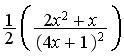We recall that (4x + 1)2 = 16x2 + 8x +1 and observe that the 2 terms on the top of the fraction bear some similarity to this expansion.

So we multiply top and bottom by 8: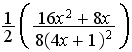Next, we add 1 to the top (to give the desired expression) and subtract one to preserve equality. On the bottom, I multiplied the 2 and 8 to give 16 outside the fraction: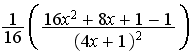Now, using our expansion, we can write the top as: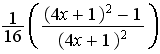This gives us simply: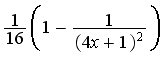So we have something that is easy to integrate. (We would set u = 4x + 1):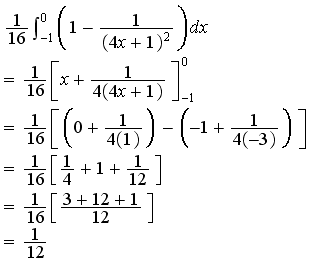OK, great - we have an answer. But didn't I say this was an impossible integral?

Tricky, yes, but impossible?

To perform the above trick, you need to have quite a bit of experience and good observation skills. What if you don't see it?

Let's have another go at substitution (which I abandoned before) and see what happens.

## Substitution method

We ignore the 1/2 to make it simpler to follow, and remember to put it back in at the end.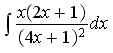We let u = 4x + 1 and this will give us

du = 4 dx

Now, to re-express the top of the fraction in terms of u, we need to find (x) and (2x + 1) in terms of u.

First, we solve u = 4x + 1 for x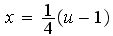Using this, we find (2x + 1):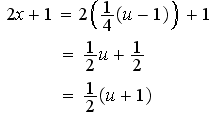So now we have all the pieces we need to integrate our fraction using substitution: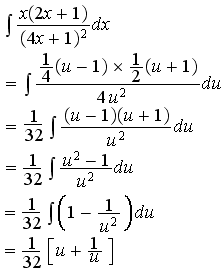Now, we consider the definitie integral (with our upper and lower limits) and remember to put back the 2 on the bottom):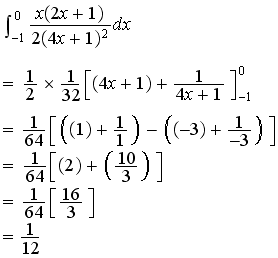So we've found an answer. But is it correct? It's the same as we got before, 1/12, but is it correct?

What's going on?

## The problem with this question

Now, for those of you who still haven't spotted why this is an impossible question, we need to consider the function and the upper and lower limits given in the question.If we graph the function we've been trying to integrate, the problem becomes immediately obvious: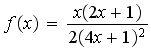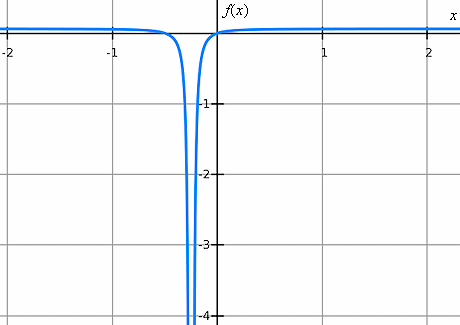In the interval -1 < x < 0, there is a discontinuity!

That is, in this example, we can never let x = -1/4, since this would give 0 on the bottom of the fraction. And of course, we can never divide by 0.

And since we can only integrate continuous functions (ones that have no holes or discontinuities), this integral is impossible!

If the limits of the integral were (say) 0 to 1, there would have been no problem at all, since the function is continuous in that interval.

## Conclusion

I have always found it very enlightening to graph the function that I'm differentiating or integrating. It can save you a lot of time wondering why things are going wrong and can help you to make sense of your answer.

And if you are a math teacher, please make sure you give your students questions that actually can be solved! (Unless of course, your aim is to trigger meaningful investigation.)

### Comment Preview

HTML: You can use simple tags like <b>, <a href="...">, etc.

To enter math, you can can either:

1. Use simple calculator-like input in the following format (surround your math in backticks, or qq on tablet or phone):
a^2 = sqrt(b^2 + c^2)
(See more on ASCIIMath syntax); or
2. Use simple LaTeX in the following format. Surround your math with $$ and $$.
$$\int g dx = \sqrt{\frac{a}{b}}$$
(This is standard simple LaTeX.)

NOTE: You can mix both types of math entry in your comment.